R for Data Science: Chapter 1

These are my solutions to the Exercises in Chapter 1 of R for Data Science.

First, I need to import the tidyverse:

library('tidyverse')
## -- Attaching packages ----------------------------------------------------------------------------------------------- tidyverse 1.3.0 --
## v ggplot2 3.3.2     v purrr   0.3.4
## v tibble  3.0.3     v dplyr   1.0.2
## v tidyr   1.1.2     v stringr 1.4.0
## v readr   1.3.1     v forcats 0.5.0
## -- Conflicts -------------------------------------------------------------------------------------------------- tidyverse_conflicts() --
## x dplyr::filter() masks stats::filter()
## x dplyr::lag()    masks stats::lag()

# Section I

Question 1: Run ggplot(data = mpg). What do you see? An empty coordinate system

ggplot(data = mpg)Question 2: How many rows are in mtcars? How many columns? 32 Rows, 11 Columns

mtcars

Question 3: What does the drv variable describe? Read the help for ?mpg to find out. The type of drive train, where f = front-wheel drive, r = rear wheel drive, 4 = 4wd

# ?mpg
# omitted code to avoid TMI

Question 4: Make a scatterplot of hwy versus cyl.

ggplot(data = mpg) +
geom_point(mapping = aes(x = cyl, y = hwy))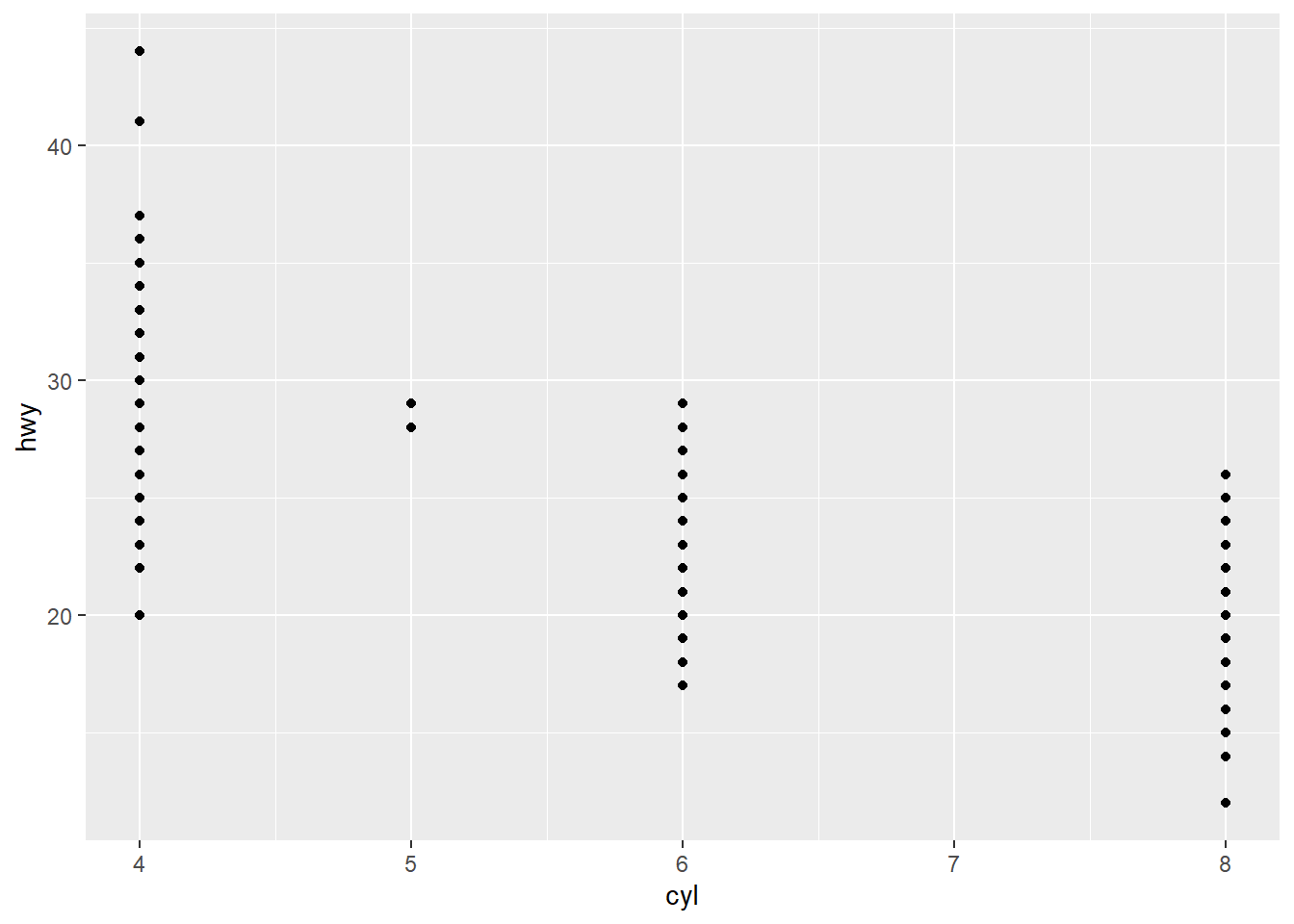Question 5: What happens if you make a scatterplot of class versus drv? Why is the plot not useful? The plot is not useful because drv is descriptive, not inferential

ggplot(data = mpg) +
geom_point(mapping = aes(x = drv, y = class))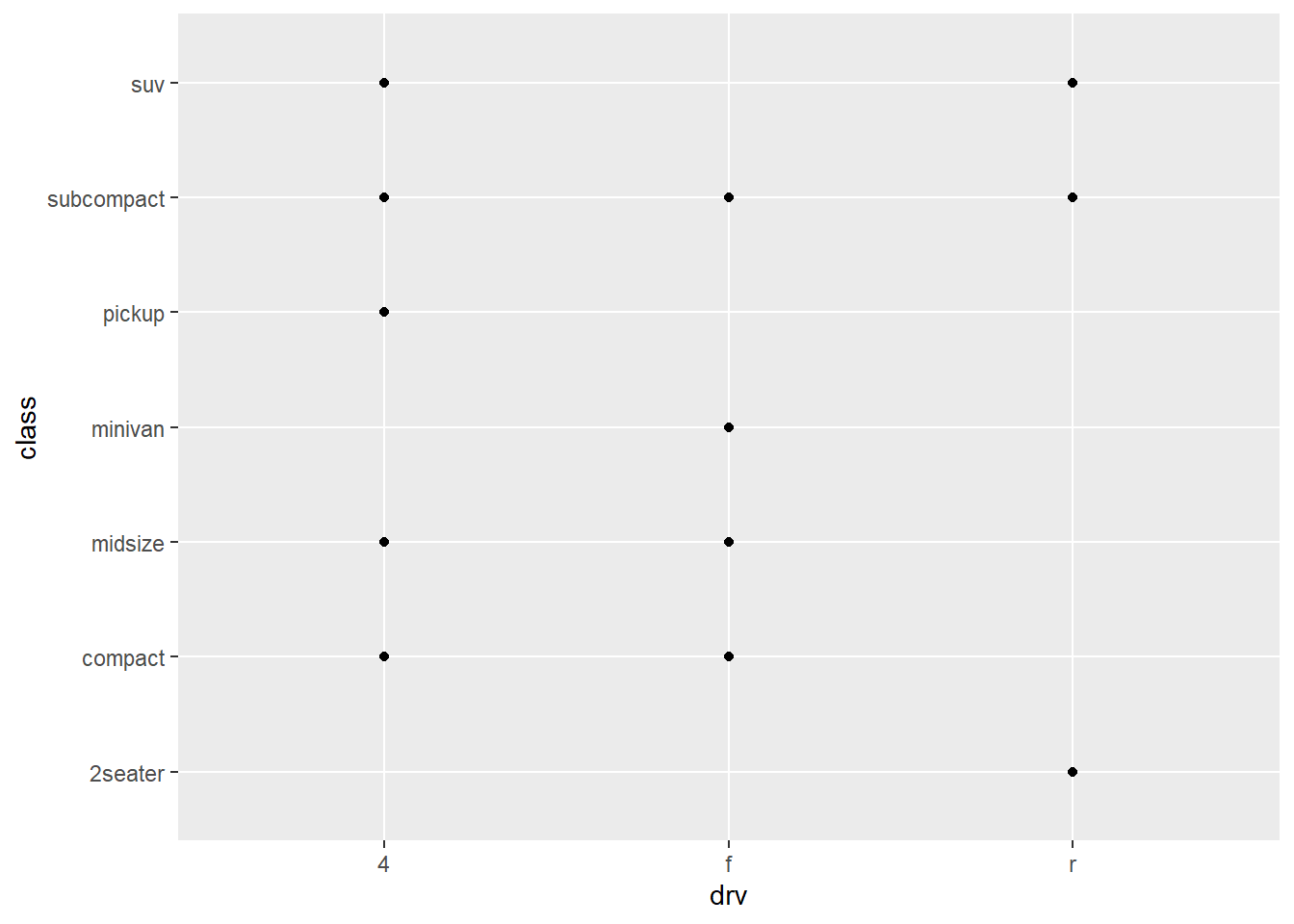# Section II

Question 1: What’s gone wrong with this code? Why are the points not blue? The manual assignment of color is not outside the aes() invocation.

ggplot(data = mpg) +
geom_point(mapping = aes(x = displ, y = hwy, color = "blue"))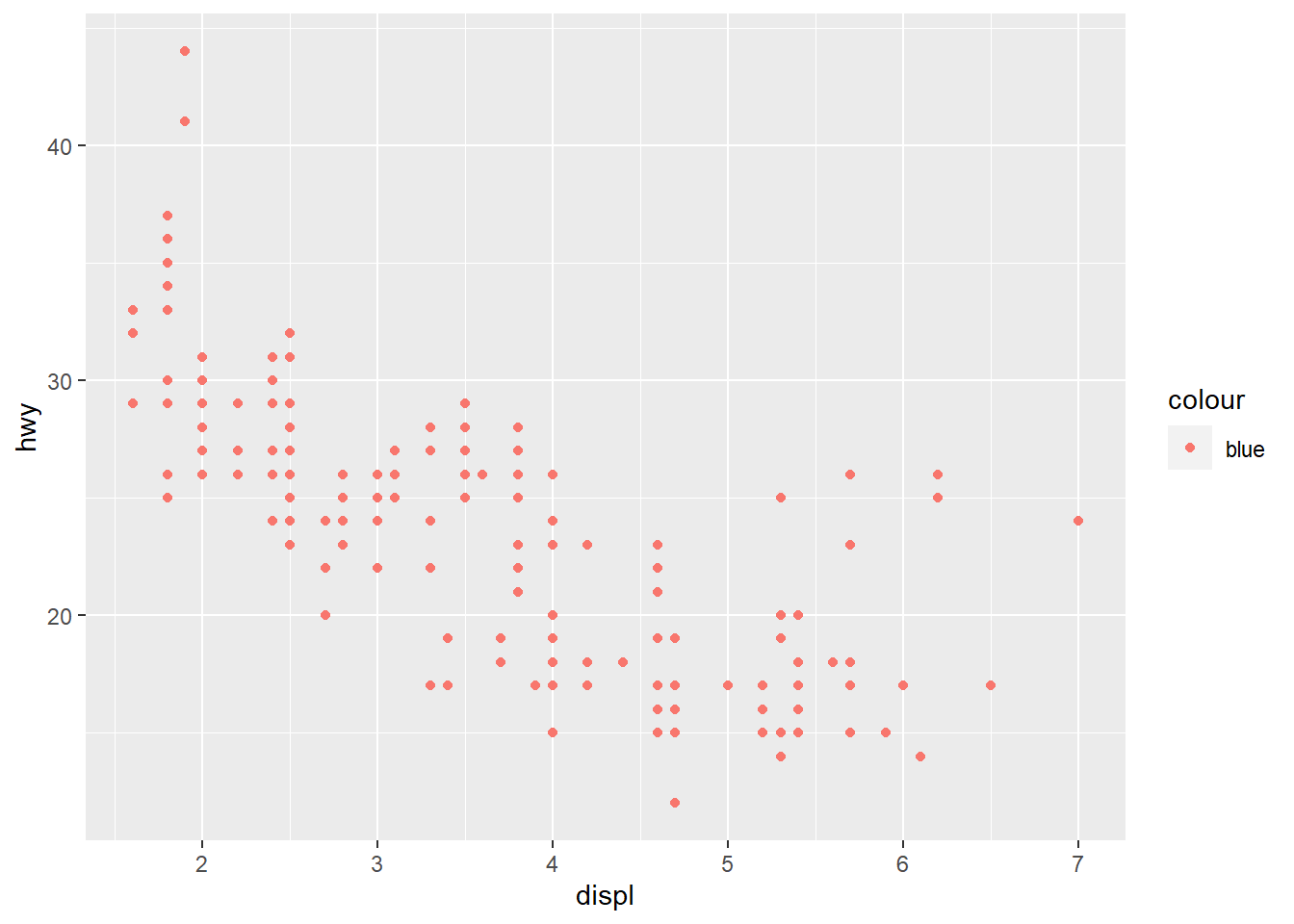Question 2: Which variables in mpg are categorical? Which variables are continuous? Categorical: manufacturer, cyl, model, trans, drv, fl, class | Continuous: disp, year, cty, hwy | cyl could be considered continuous if it were likely the values would differ more than they do, but as most cars have 4, 6, or 8 cylinders in this dataset, I classify it as categorical.

Question 3: Map a continuous variable to color, size, and shape. How do these aesthetics behave differently for categorical versus continuous variables? For shape, an error will occur: Error: A continuous variable can not be mapped to shape. For size and color, no error will be thrown. The color will be put on a gradient scale, and the size will bucket values into one of 6 buckets whose size corresponds to some value within the range of the dataset

ggplot(data = mpg) +
geom_point(mapping = aes(x = displ, y = hwy, color=year, size=cty)) #I removed shape=year here, to avoid the error.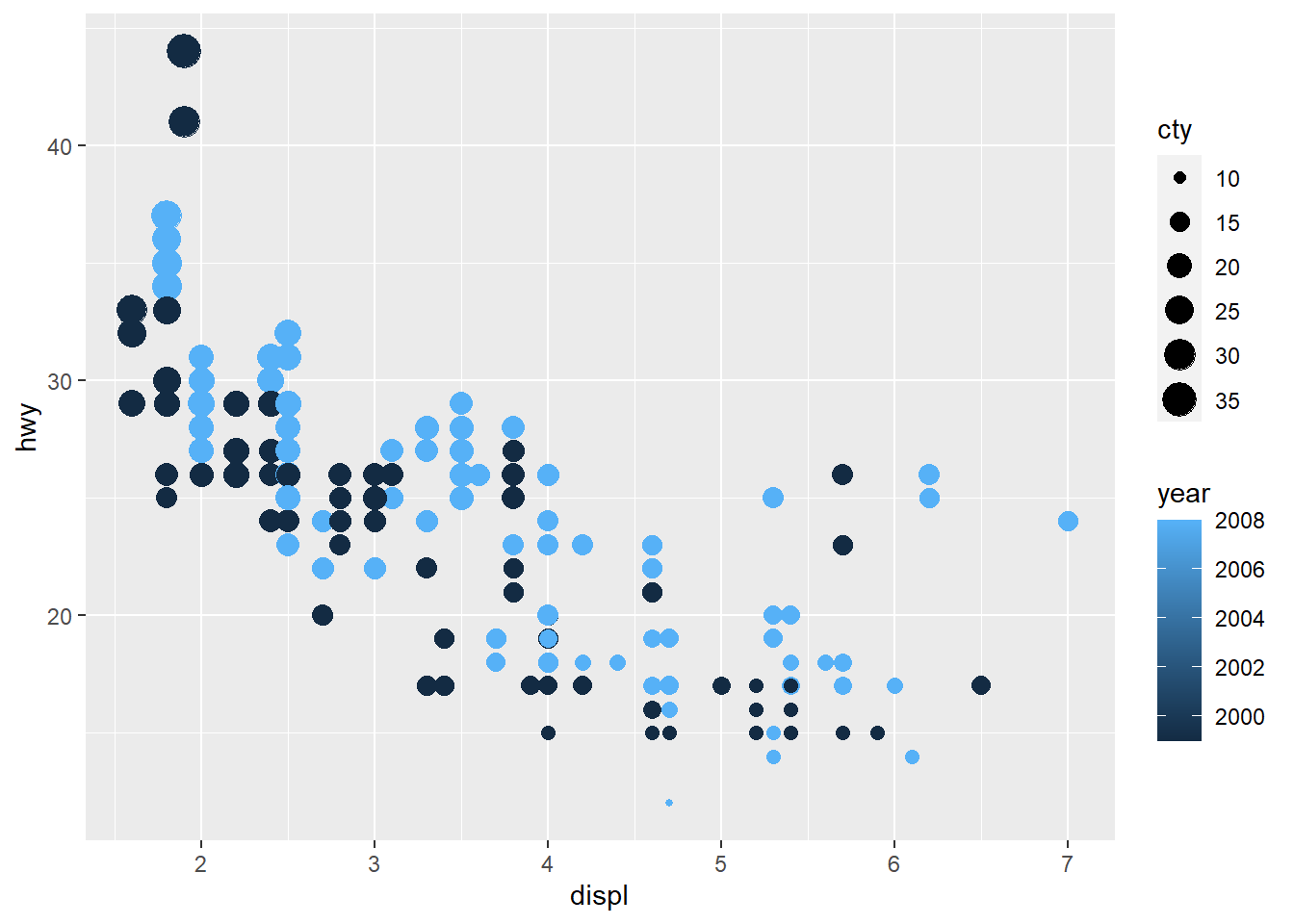Question 4: What happens if you map the same variable to multiple aesthetics? It will work, although because size already buckets that value, the color then becomes redundant.

ggplot(data = mpg) +
geom_point(mapping = aes(x = displ, y = hwy, color=cty, size=cty))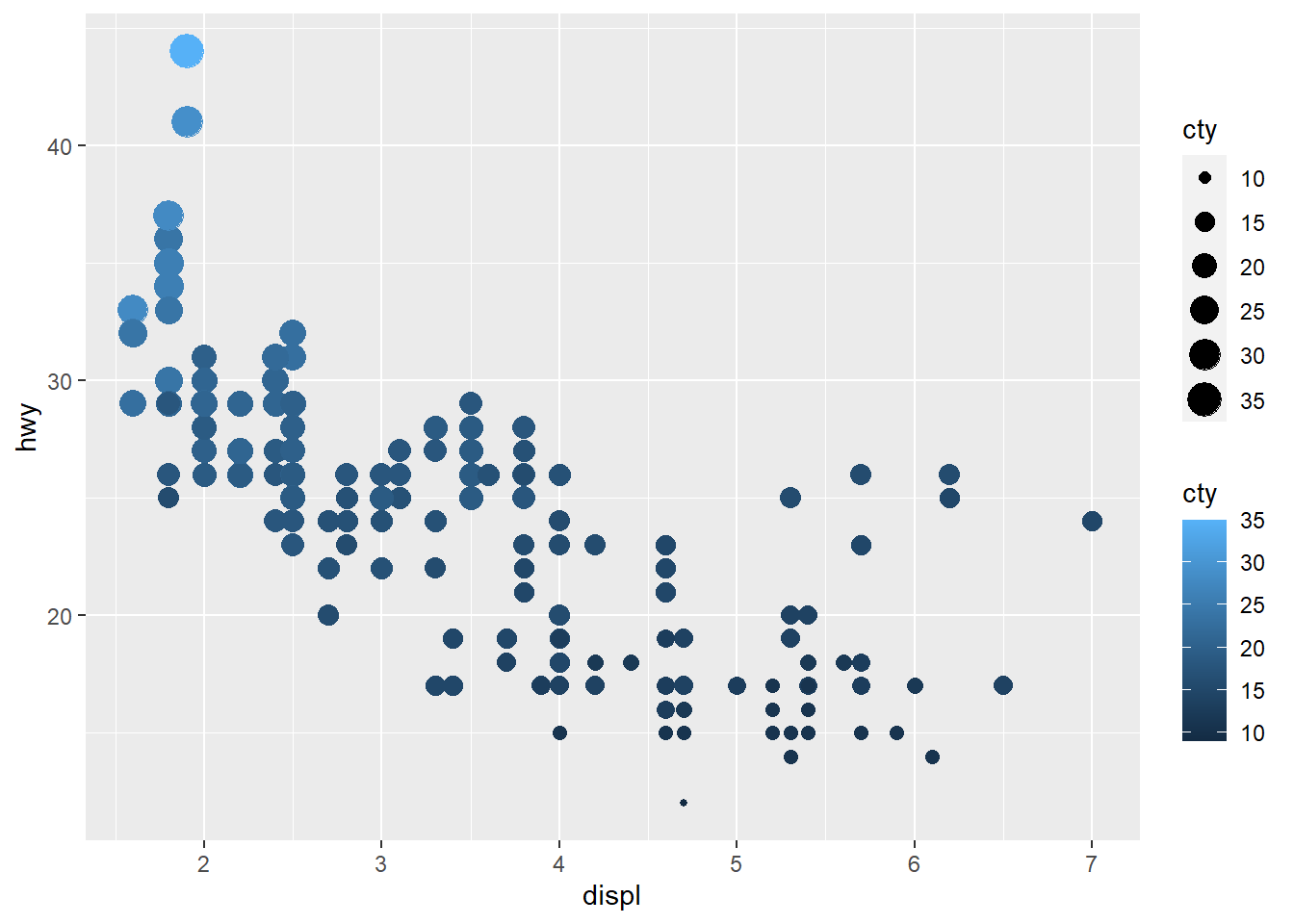Question 5: What does the stroke aesthetic do? What shapes does it work with? Modifies the width of the border. Works with any shapes that have a border, like 21-24.

# ?geom_point
# omitted code to avoid TMI

Question 6: What happens if you map an aesthetic to something other than a variable name, like aes(color = displ < 5)? Because displ < 5 results in a boolean value, each observation will be colored by the result of checking whether or not displ has a value less than 5.

ggplot(data = mpg) +
geom_point(mapping = aes(x = displ, y = hwy, color = displ < 5))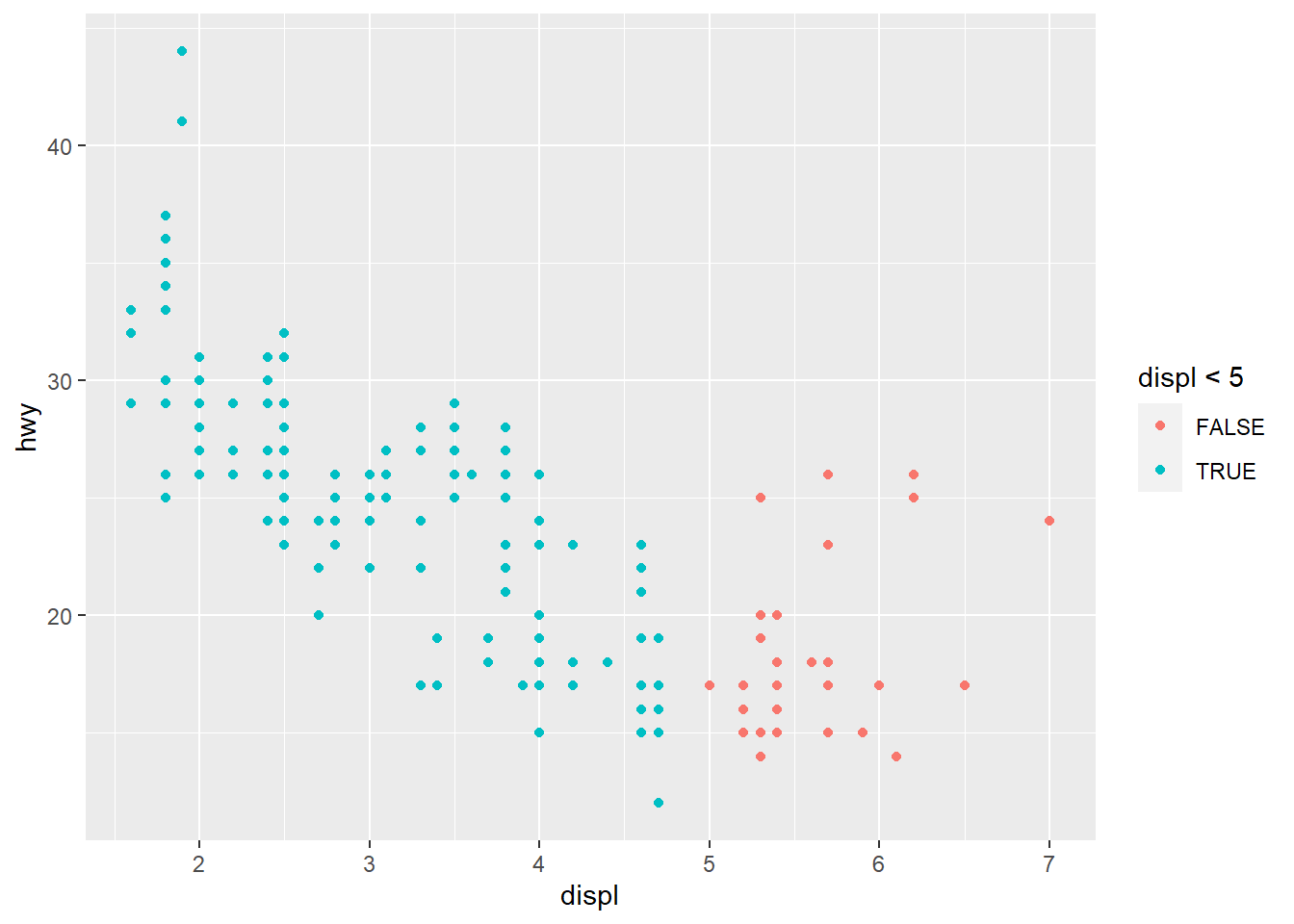# Section III

Question 1: What happens if you facet on a continuous variable? You get a plot for each value of that variable

ggplot(data = mpg) +
geom_point(mapping = aes(x = displ, y = hwy)) +
facet_wrap(~ displ, nrow = 2)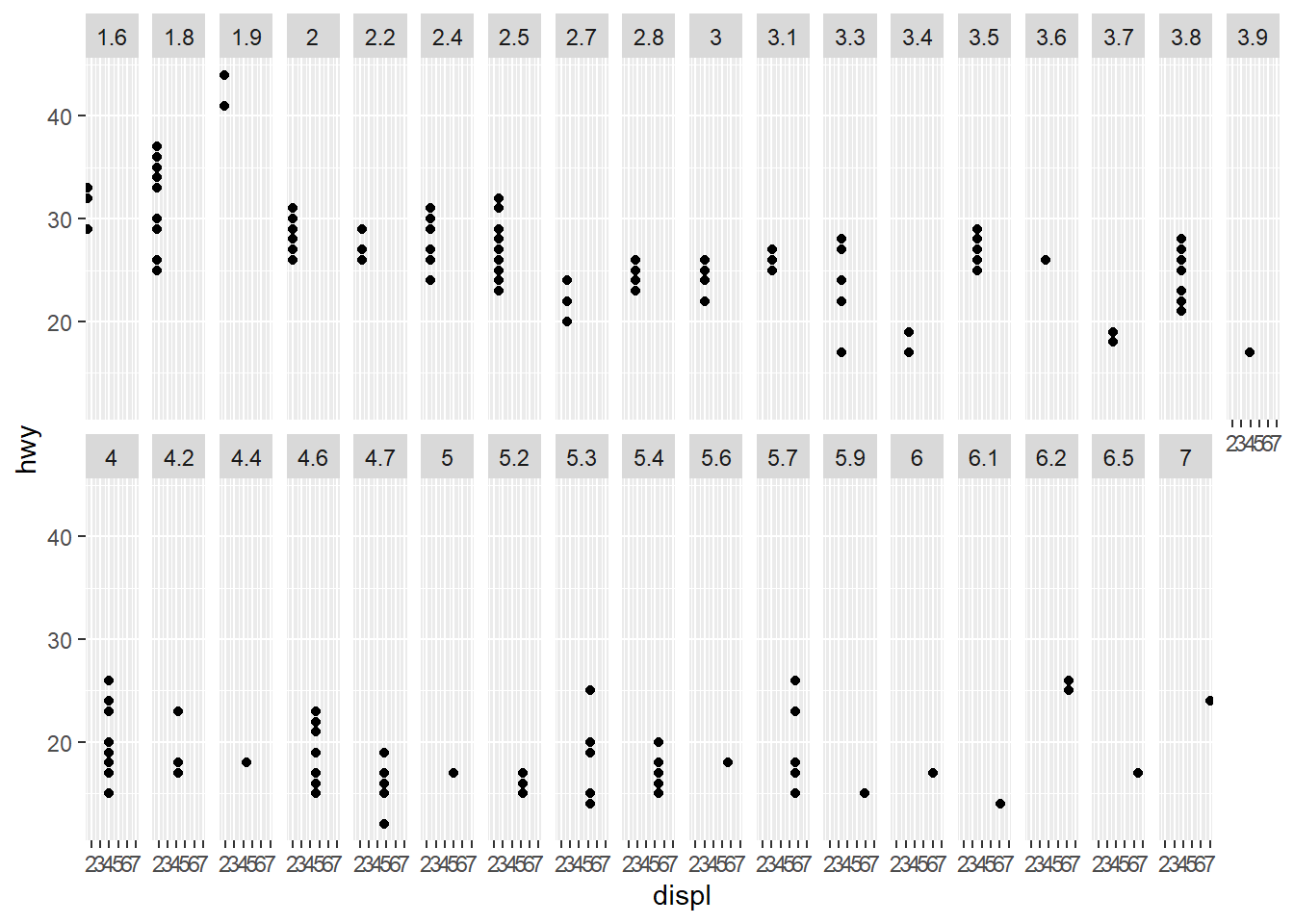Question 2: What do the empty cells in a plot with facet_grid(drv ~ cyl) mean? It means there are no observations within the mpg data set where the value for drv and the value for cyl match the criteria. In other words, there are no 4wd, 5-cyl vehicles.

ggplot(data = mpg) +
geom_point(mapping = aes(x = displ, y = hwy)) +
facet_grid(drv ~ cyl)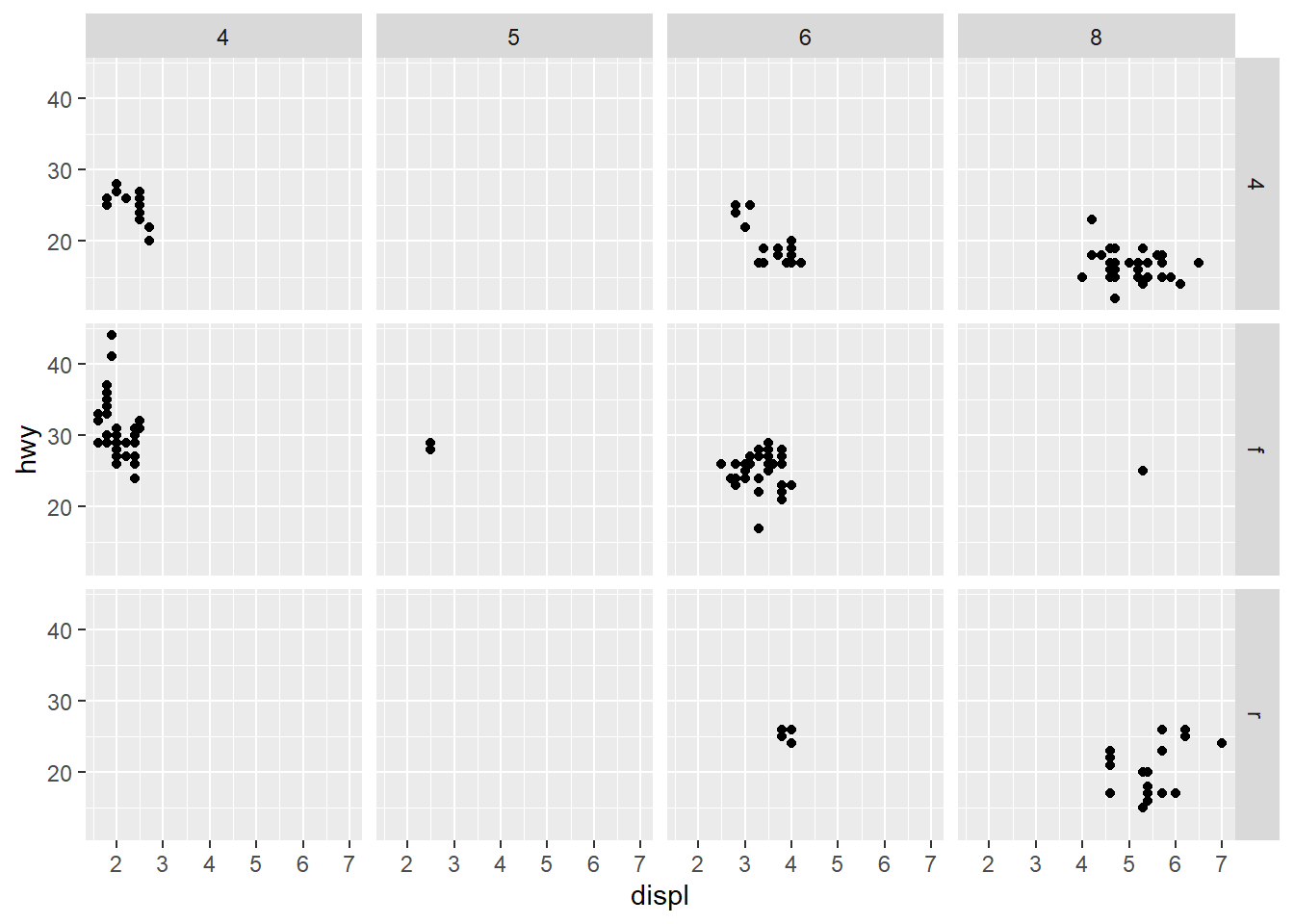Question 3: What does the following code make? What does . do? If placed after the categorical variable, it splits the plots horizontally so that each value of that category has its own y-axis while sharing the x axis. If placed before the categorical variable, it splits the plots vertically so that each value has its own x-axis while sharing the y axis.

ggplot(data = mpg) +
geom_point(mapping = aes(x = displ, y = hwy)) +
facet_grid(drv ~ .)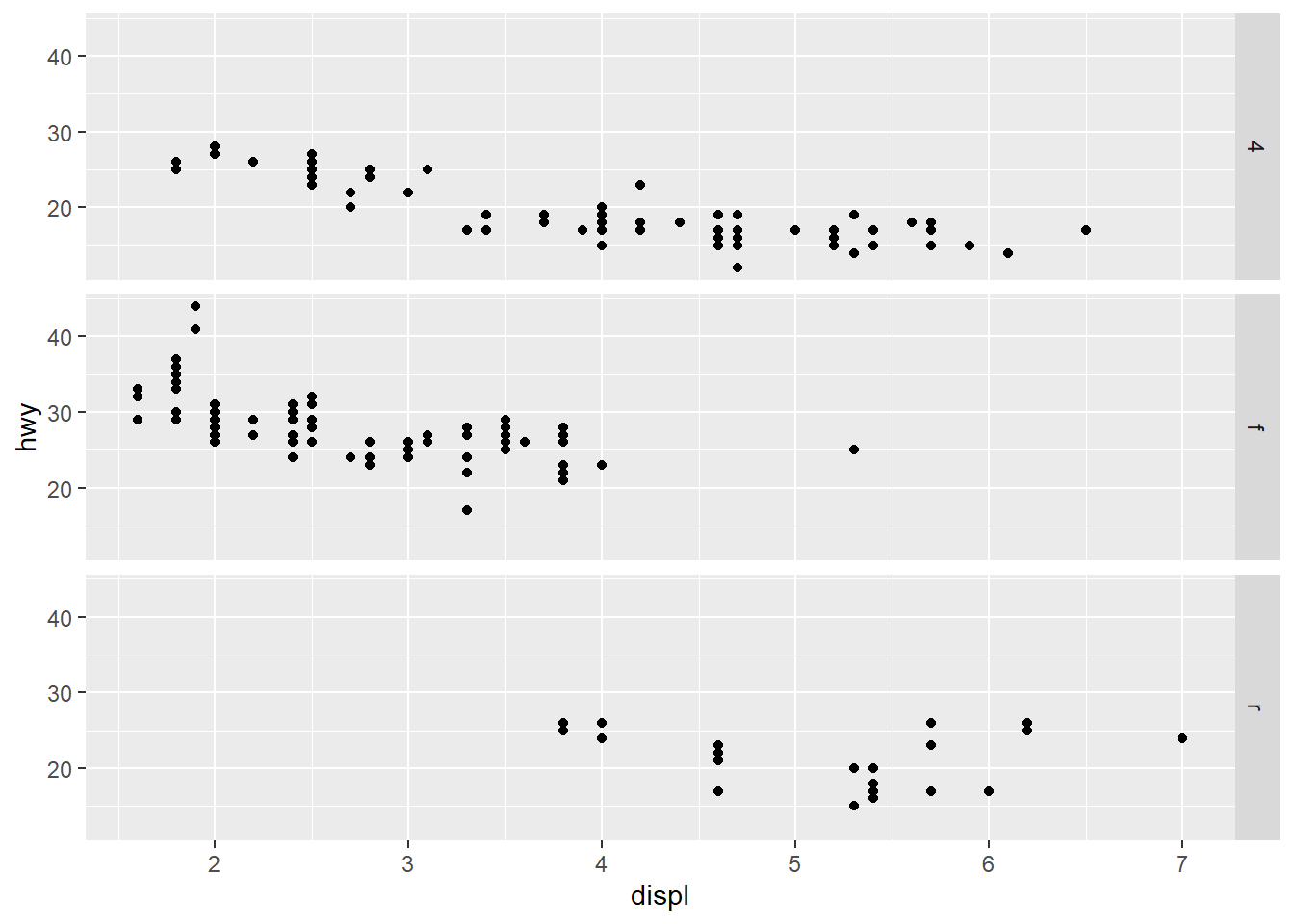ggplot(data = mpg) +
geom_point(mapping = aes(x = displ, y = hwy)) +
facet_grid(. ~ cyl)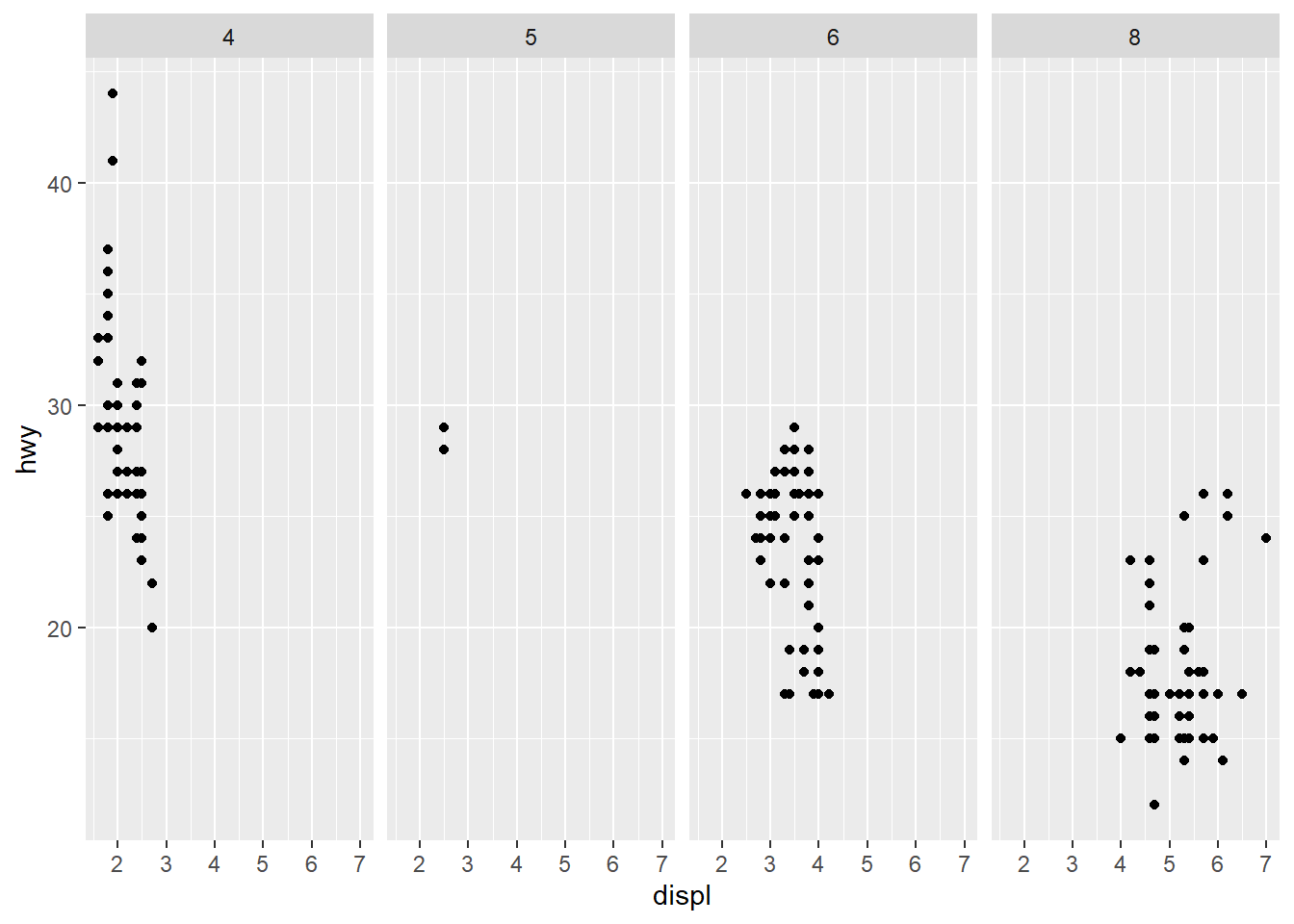Question 4: Take the first faceted plot in this section. What are the advantages to using faceting instead of the color aesthetic? What are the disadvantages? How might the balance change if you had a larger dataset? The advantages to using faceting instead of coloring is that it isolates the observations to their own plot within some category. This makes it a bit quicker to identify all observations within a certain criteria (i.e. compact cars). The disadvantages of this are that it is trickier to see similarities between two different classes of vehicles using faceting than it would be with the color aesthetic. By using color, you’d see these observations plotted on top of one another in the same plot, so you could see that compact and subcompact vehicles share very similar patterns when it comes to displ/hwy much easier than you can with faceting. If you had a much larger dataset, it would depend on how much variation there is within the data. If we had 10,000 more vehicles in each categorical class, it may be difficult to tell by coloring that compact and subcompact vehicles have some similarities - but with faceting, the basic shape of the data would tell us this.

ggplot(data = mpg) +
geom_point(mapping = aes(x = displ, y = hwy)) +
facet_wrap(~ class, nrow = 2)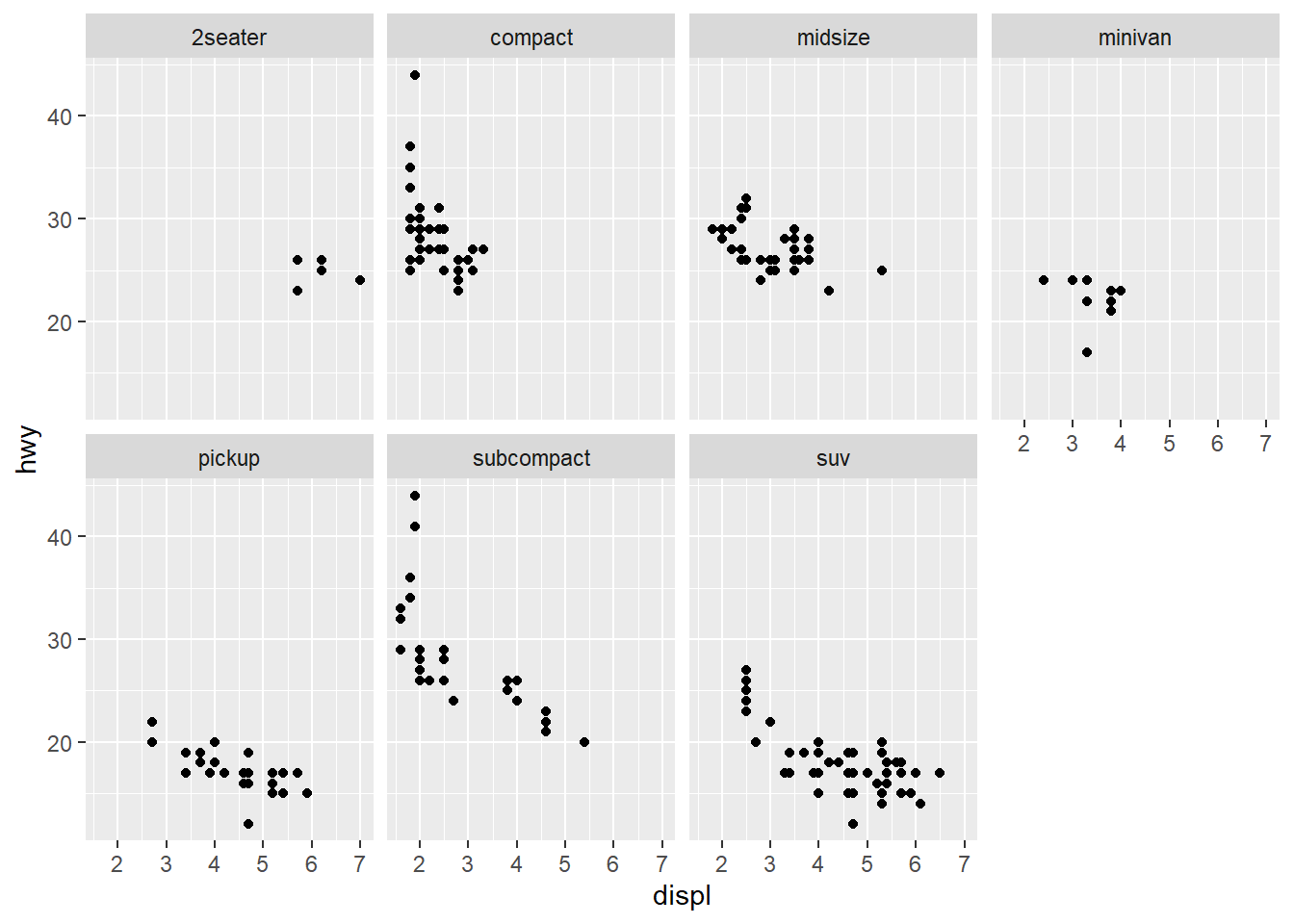Question 5: Read ?facet_wrap. What does nrow do? What does ncol do? What other options control the layout of the individual panels? Why doesn’t facet_grid() have nrow and ncol variables? nrow allows you to set the number of rows in your facet wrap, while ncol lets you define the number of columns. facet_grid() does not have these because it will create as many as necessary to match the dataset’s length and width.

# ?facet_wrap
# ommitted to avoid TMI

Question 6: When using facet_grid() you should usually put the variable with more unique levels in the columns. Why? This makes it easier to compare each level’s value of the dependent variable (the y-axis). Another reason (that could be fixed by a matter of extra arguments to facet_grid() is that the default height of the grid has a smaller value than the default width.